# Linear Regression: Least Squares Fit of a Straight Line to a Set of Points. Includes a Proof that the Total Variance can be Divided into Component Parts, and also Includes a Derivation of the Correlation Coefficient, Explaining its Meaning

We want to fit a straight line of the form: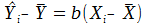(Eq. 1) or, alternatively,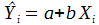(Eq. 2) to a set of data points.

Using the least squares method, we want to minimize the function: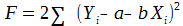(Eq. 3).

Taking partial derivatives and setting them to zero, we get: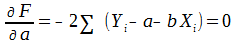(Eq. 4).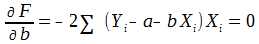(Eq. 5).

Solving these equations, we get: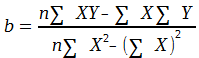(Eq. 6), and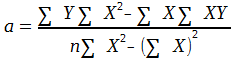(Eq. 7),

or,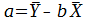(Eq. 8).

In the following, we will assume that the number of points, n, in our data set is
quite large, say, >= 100.
So to calculate variances we will simply divide sums
by n (rather than n-1 or n-2 to take into account degrees of freedom).
Consequently, the variances we will calculate are only close approximations.

Using the values of a and b that we calculated above to minimize the F function,
we have:So,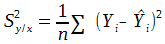(Eq. 9),

where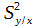is the part of the total variance,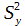that is left unexplained by the regression.

The other part of the total variance is variance explained by the regression,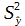The explained and unexplained variances should add up to the total variance, i.e., that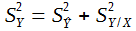(Eq. 10), and we will now prove that.

Equation 10 can also be written as: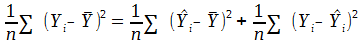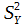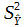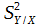We start the proof with: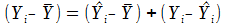(identity).

Squaring both sides and summing, we get: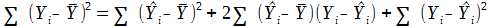.

So the theorem is true if: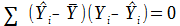.

Or equivalently if: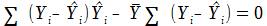(Eq. 11).

(A)                       (B)

From Equation 4, since we are using the optimum values for a and b, we know that: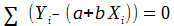, and, substituting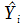for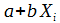, that means that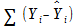is zero. So (B) above is true.
From equation 5, we know that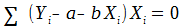. Substituting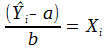, we get: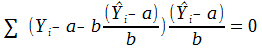Simplifying, we get: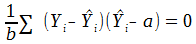,

and: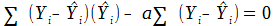. Since (B) , above, is zero, that means that (D) is zero,

(C)                            (D)

which in turn means that (C) is zero. And (C) is the same as (A), so (A) is zero.
Then, because (A) and (B) are both zero, equation 11 is zero. This proves
equation 10, that: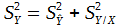, i.e., that the total variance can be separated into two
non-overlapping components, the variance explained and the variance
unexplained by the regression, that added together are equal to the total variance.

Now we will look at the relationship between the total variance and the unexplained variance and define a correlation coefficient, r.

Squaring equation 1,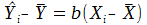, we get: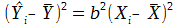.

Summing over n yields: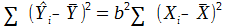, which means that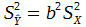.

Substituting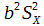forin Equation 10, we have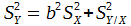, or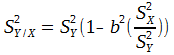A standard equation for the variance of a sample is: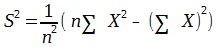.

And since, from equation 6,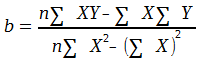, then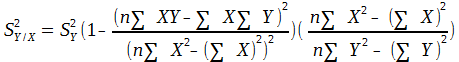, or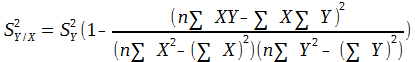The rightmost term on the right hand side of this equation is the square of the correlation coefficient, r.

So,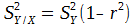(Eq. 12).

From equation 10, we know that, or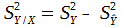.

Substituting into equation 12,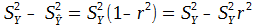.

So,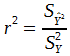In words,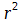is the ratio of the variance explained by the regression of Y on X to the total variance. This, in my opinion provides a better definition of the correlation coefficient than its usual definition as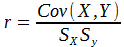.